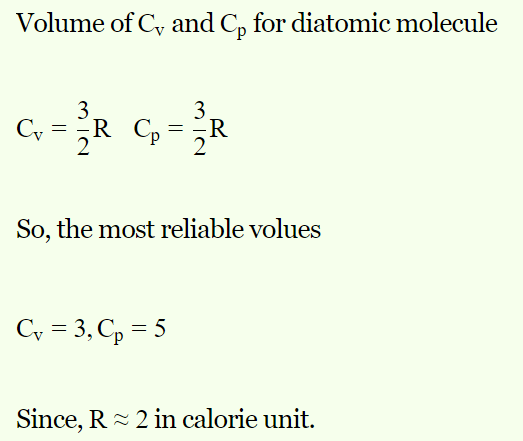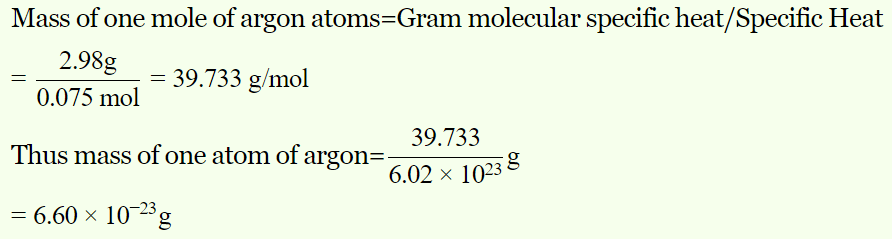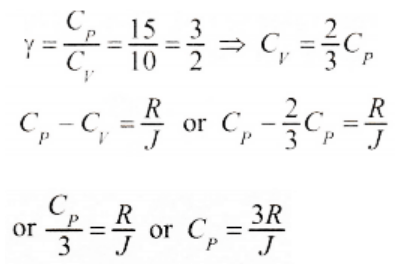## Kinetic Theory Questions and Answers Part-18

1. The specific heat of an ideal gas is
a) Proportional to T
b) Proportional to $T^{2}$
c) Proportional to $T^{3}$
d) Independent of T

Explanation: The specific heat of an ideal gas is independent of T

2. Molar specific heat at constant volume is $C_{V}$ for a monoatomic gas is
a) $\frac{3}{2}R$
b) $\frac{5}{2}R$
c) 3R
d) 2R

Explanation: Molar specific heat at constant volume is $C_{V}$ for a monoatomic gas is $\frac{3}{2}R$

3. The following sets of values for $C_{V}$  and $C_{P}$  of a gas has been reported by different students. The units are cal/gm-mole-K. Which of these sets is most reliable
a) $C_{V}=3,C_{P}=5$
b) $C_{V}=4,C_{P}=6$
c) $C_{V}=3,C_{P}=2$
d) $C_{V}=3,C_{P}=4.2$

Explanation:4. The specific heat at constant volume for the monoatomic argon is 0.075 kcal/kg-K, whereas its gram molecular specific heat $C_{V}$  = 2.98 cal/mole/K . The mass of the argon atom is
a) $6.60\times 10^{-23}gm$
b) $3.30\times 10^{-23}gm$
c) $2.20\times 10^{-23}gm$
d) $13.20\times 10^{-23}gm$

Explanation:5. Supposing the distance between the atoms of a diatomic gas to be constant, its specific heat at constant volume per mole (gram mole) is
a) $\frac{5}{2}R$
b) $\frac{3}{2}R$
c) R
d) $\frac{1}{2}R$

Explanation: Supposing the distance between the atoms of a diatomic gas to be constant, its specific heat at constant volume per mole (gram mole) is $\frac{5}{2}R$

6. For a certain gas, the ratio of specific heats is given to be $\gamma=1.5$ . For this gas
a) $C_{V}=\frac{3R}{J}$
b) $C_{P}=\frac{3R}{J}$
c) $C_{V}=\frac{5R}{J}$
d) $C_{P}=\frac{5R}{J}$

Explanation:7.The specific heats at constant pressure is greater than that of the same gas at constant volume because
a) At constant pressure work is done in expanding the gas
b) At constant volume work is done in expanding the gas
c) The molecular attraction increases more at constant pressure
d) The molecular vibration increases more at constant pressure

Explanation: The specific heats at constant pressure is greater than that of the same gas at constant volume because at constant pressure work is done in expanding the gas

8. The specific heat of a gas
a) Has only two values $C_{p}$  and $C_{V}$
b) Has a unique value at a given temperature
c) Can have any value between 0 and $\infty$
d) Depends upon the mass of the gas

Explanation: The specific heat of a gas can have any value between 0 and $\infty$

9. The molar specific heat at constant pressure for a monoatomic gas is
a) $\frac{3}{2}R$
b) $\frac{5}{2}R$
c) $\frac{7}{2}R$
d) 4 R

Explanation: The molar specific heat at constant pressure for a monoatomic gas is $\frac{5}{2}R$
10. For a gas if $\gamma=1.4$ , then atomicity, $C_{p}$  and $C_{V}$  of the gas are respectively
a) Monoatomic, $\frac{5}{2}R,\frac{3}{2}R$
b) Monoatomic, $\frac{7}{2}R,\frac{5}{2}R$
c) Diatomic, $\frac{7}{2}R,\frac{5}{2}R$
d) Triatomic,$\frac{7}{2}R,\frac{5}{2}R$
Explanation: Diatomic, $\frac{7}{2}R,\frac{5}{2}R$